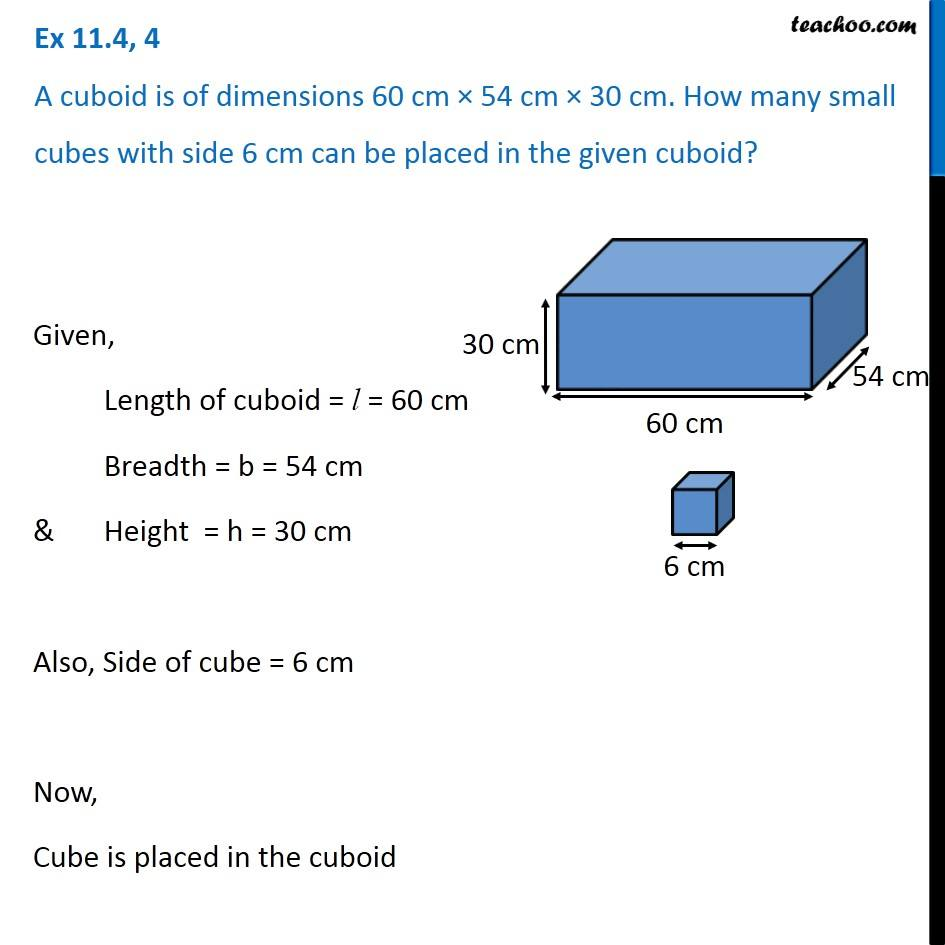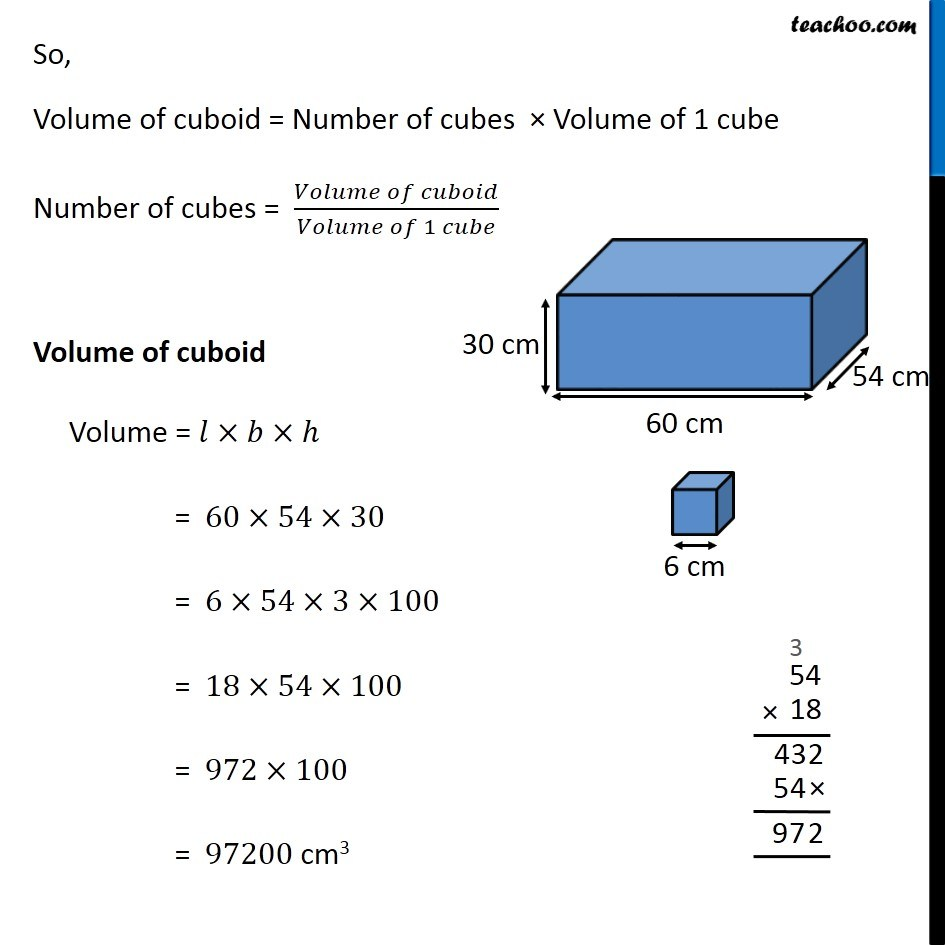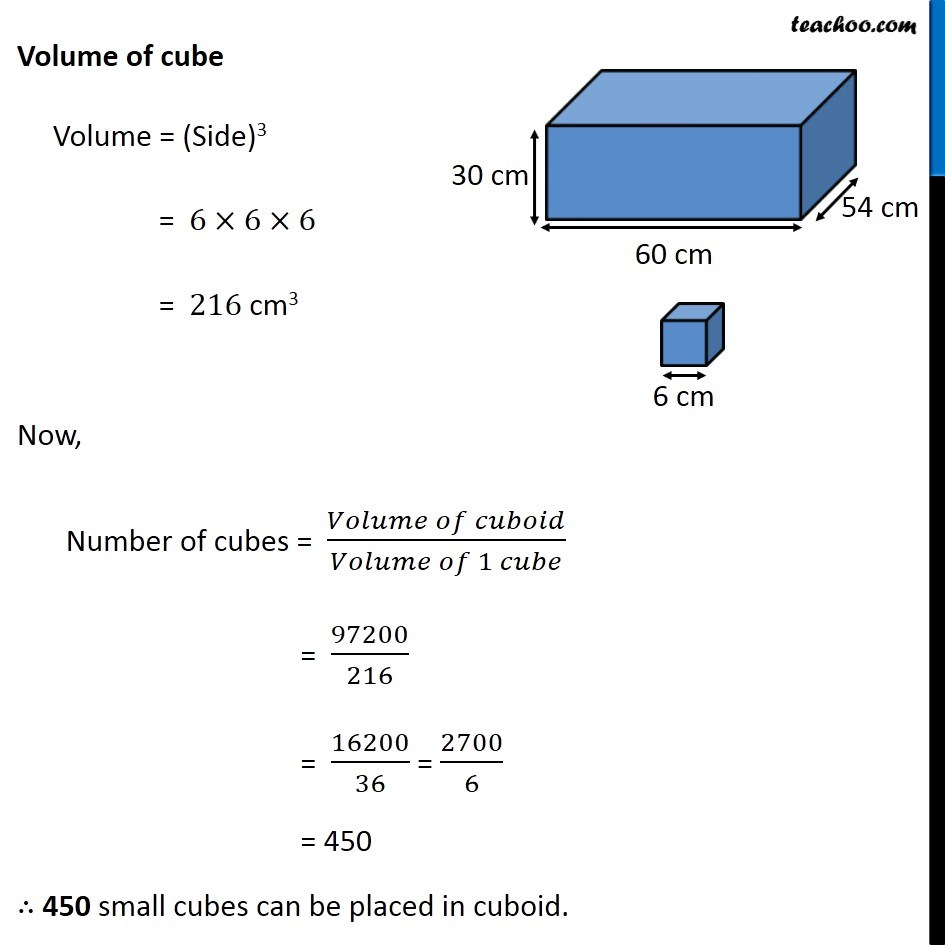Volume of Cuboid

Chapter 11 Class 8 Mensuration
Concept wiseIntroducing your new favourite teacher - Teachoo Black, at only ₹83 per month

### Transcript

Ex 11.4, 4 A cuboid is of dimensions 60 cm × 54 cm × 30 cm. How many small cubes with side 6 cm can be placed in the given cuboid? Given, Length of cuboid = l = 60 cm Breadth = b = 54 cm & Height = h = 30 cm Also, Side of cube = 6 cm Now, Cube is placed in the cuboid So, Volume of cuboid = Number of cubes × Volume of 1 cube Number of cubes = (𝑉𝑜𝑙𝑢𝑚𝑒 𝑜𝑓 𝑐𝑢𝑏𝑜𝑖𝑑)/(𝑉𝑜𝑙𝑢𝑚𝑒 𝑜𝑓 1 𝑐𝑢𝑏𝑒) Volume of cuboid Volume = 𝑙×𝑏×ℎ = 60×54×30 = 6×54×3×100 = 18×54×100 = 972×100 = 97200 cm3 Volume of cube Volume = (Side)3 = 6×6×6 = 216 cm3 Now, Number of cubes = (𝑉𝑜𝑙𝑢𝑚𝑒 𝑜𝑓 𝑐𝑢𝑏𝑜𝑖𝑑)/(𝑉𝑜𝑙𝑢𝑚𝑒 𝑜𝑓 1 𝑐𝑢𝑏𝑒) = 97200/216 = 16200/36 = 2700/6 = 450 ∴ 450 small cubes can be placed in cuboid.